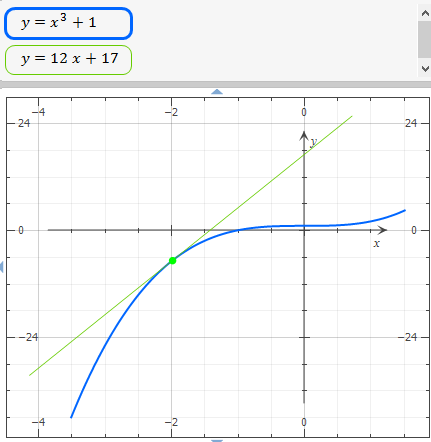# a. Find the slope of this equation y = x^3+1 at the point P(-2, -7) by finding the limiting value...

## Question:

a. Find the slope of this equation y = x{eq}^3 {/eq}+1 at the point P(-2, -7) by finding the limiting value of the slope of the secants through P.

b. Find an equation of the tangent line to the curve at P(-2, -7)

## Tangent line

In calculus, a tangent line is a straight line that touches the function at only one point, to find it, we need a function with one independent variable, its first derivative, and one point.

The function is:

{eq}\displaystyle \ f(x) \, = \, x^3+1 \\ {/eq}

a. Find the slope

{eq}\displaystyle \lim_{ h \; \rightarrow \; 0 } \frac{ \ f(x+h)- \ f(x) \, }{ h } \, = \, \displaystyle \lim_{ h \; \rightarrow \; 0 } \frac{ \left( (x+h)^3+1 \right)- \left( x^3+1 \right) }{ h } \\ \displaystyle \lim_{ h \; \rightarrow \; 0 } \frac{ \left( (x+h)^3+1 \right)- \left( x^3+1 \right) }{ h } \; = \; \displaystyle \lim_{ h \; \rightarrow \; 0 } \frac{ x^3+3hx^2+3xh^2+h^3+1-x^3-1 }{h } \\ \displaystyle \lim_{ h \; \rightarrow \; 0 } \frac{ 3hx^2+3xh^2+h^3 }{h } \; = \; \left( \frac{ h }{ h } \right) \left( 3x^2 \right) \\ \displaystyle \lim_{ h \; \rightarrow \; 0 } \frac{ 3hx^2+3xh^2+h^3 }{h } \; = \; 3x^2 \\ {/eq}

Then, the slope of this equation, at the point P {eq}(-2,-7) {/eq} is: {eq}\displaystyle \ f'(x) \; = \; 3x^2 \; \Rightarrow \; \ f'(-2) \; = \; 3(-2)^2 \\ \displaystyle \ f'(-2) \; = \; 12 \\ {/eq}

b. Find an equation of the tangent line to the curve at P(-2, -7)

Tangent line equation for any point {eq}\displaystyle (c,f(c)) {/eq} is:

{eq}\displaystyle y \; = \; f'(c)(x-c)+ \ f(c) \\ {/eq}

so,

{eq}\displaystyle y \; = \; f'(-2)(x-(-2))+ \ f(-2) \\ \displaystyle y \; = \; \left( 12 \right) (x+2 )-7 \\ \displaystyle y \; = \; 12x+24-7 \\ \displaystyle y \; = \; 12x +17 \\ {/eq}

Graph